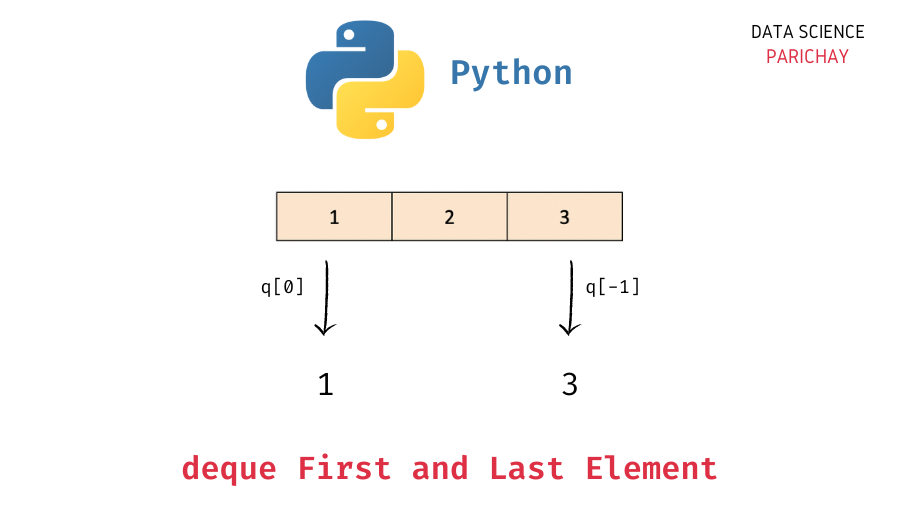# Python – Get the First and Last Element in a Deque

In this tutorial, we will look at how to get the first and the last element in a deque in Python (without deleting any elements) with the help of some examples.

## `deque` in Python

The `collections` module in Python comes with a `deque` class that you can use to implement a stack or queue data structure in Python. Deques support thread-safe, memory efficient appends and pops from either side of the deque with approximately the same O(1) performance in either direction.

In simple terms, you can think of a `deque` in Python as a list-like data structure but with efficient insertion and removal of items from either end (left and right). This makes them very useful when implementing a stack or queue functionality in Python.

## How to get the first and the last element in a deque in Python?

Deques in Python make it very easy to add and remove elements from either end.

You can use the `pop()` function to get the last element in the deque and the `popleft()` function to get the first element in the deque but these methods modify the deque by removing the concerned element.

What if you just want to get the first and/or the last element without modifying the deque object?

Similar to lists, deques in Python are indexed starting from 0 and thus you can use the index 0 to get the first element and the index -1 to get the last element in a deque.

The following is the syntax –

📚 Data Science Programs By Skill Level

Introductory

Intermediate ⭐⭐⭐

🔎 Find Data Science Programs 👨‍💻 111,889 already enrolled

Disclaimer: Data Science Parichay is reader supported. When you purchase a course through a link on this site, we may earn a small commission at no additional cost to you. Earned commissions help support this website and its team of writers.

```# get the first element in the deque "queue"
queue
# get the last element in the deque "queue"
queue```

This is similar to how you’d get the first and last values in any ordered iterable in Python.

## Examples

Let’s now look at some examples of using the above syntax

First, let’s create a deque with some elements.

```from collections import deque

# create a deque
queue = deque([1, 2, 3])
# print the deque
print(queue)```

Output:

`deque([1, 2, 3])`

Here, we imported the `deque` class from the `collections` module and created a deque object, `queue` with some numbers. You can see that the deque contains three values 1, 2, and 3.

### Example 1 – Get the first element in a deque

To get the first element in a deque, access the value at the index 0 using `[]` notation.

```# first value in the deque
print(queue)```

Output:

`1`

We get the first value in the deque, `queue` as `1`.

### Example 2 – Get the last element in a deque

To get the last element in a deque, access the value at the last index (using a negative index can be helpful here). The index `-1` represent the index of the last value.

```# last value in the deque
print(queue[-1])```

Output:

`3`

We get the last value in the deque, `queue` as `3`.

You might also be interested in –

•